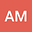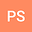Time-space Jacobi pseudospectral simulation of multidimensional Schrodinger equation
••• AVINASH MITTAL,
• Parnika shrivastava
AVINASH MITTAL
Indian Institute of Information Technology Design and Manufacturing Jabalpur
Author ProfileParnika shrivastava
NIT Hamirpur
Author Profile## Abstract

In this paper, the authors investigate the interaction of soliton waves for multidimensional nonlinear Schrodinger equation (NSE) using time-space Jacobi pseudospectral method. The proposed method is established in both time and space to approximate the solutions and to prove the stability analysis for the equations. Using the Jacobi derivatives matrices the given problem is reduced to a system of nonlinear algebraic equations, which will be solved using Newton’s Raphson method. For numerical experiments, the method is tested on a number of different examples to study the behavior of interaction of two and more than two soliton, single soliton. Moreover, numerical solutions are demonstrated to justify the theoretical results and confirm the expected convergence rate. Comparison of numerical and exact solution is depicted in the form of figures and tables.